## Differential equations

Differential equations are called partial differential equations (PDE) or Ordinary differential equations (ODE), whether they are included or not partial derivatives. A solution (or special solution) of the differential equation of order n defines a function and has different times on one Domain D has the property that holds the functional equation obtained by substituting the function and its n derivatives into the differential equation D.

The differential equations in the form,

Equation 1.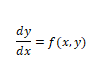Where f is given and is an unknown function of y. When found to be continuous over some interval, we found a general solution y(x) by integration. In section, We Solved Ordinary differential equations for the type of first order.

A first-order differential equation is an equation in which ƒ(x, y) is a function of two variables defined on a region in the XY-plane. The equation is of the first order because it contains only the first derivative dy/dx.
y' = f(x,y) andall these are equivalent to equation 1.

Let's take a look at how to Plot first-order differential equations in Python. The list of Different equations that we solve in Python given below. We discuss these equations one by one in an easy way.

## 1. Solving Ordinary differential equation of order first using Eq.```from scipy.integrate import odeint
import numpy as np
from matplotlib import pyplot as plt
def f(y,x):
return x
y0 = 1xs = np.arange(0,1,0.1)
ys = odeint(f,y0,xs)
plt.plot(xs,ys,'-')
plt.plot(xs,ys,'ro')
plt.xlabel('x values')
plt.ylabel('y values')
plt.title('Differential equation: dy/dx=x, y(0)=1, xrange=[0,1]')
plt.show()```
`Output:`2. Solving Ordinary differential equation of order first using Eq.```from scipy.integrate import odeint
import numpy as np
from matplotlib import pyplot as plt
def f(y,x):
return y/x
y1 = 1xs = np.arange(1,10,1)
ys = odeint(f,y1,xs)
plt.plot(xs,ys,'-')
plt.plot(xs,ys,'ro')
plt.xlabel('x values')
plt.ylabel('y values')
plt.title('Differential equation: dy/dx=y/x, y(1)=1')
plt.show()```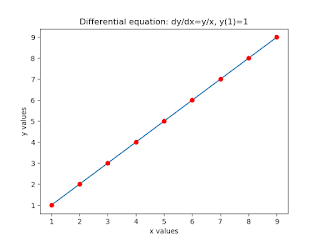3. Solving Ordinary differential equation of order first using Eq.```from scipy.integrate import odeint
import numpy as np
from matplotlib import pyplot as plt
def f(y,x):
return (x*y/(np.sqrt(y**2+x)))
y0 = 1xs = np.arange(0,5,0.25)
ys = odeint(f,y0,xs)
plt.plot(xs,ys,'-')
plt.plot(xs,ys,'ro')
plt.xlabel('x values')
plt.ylabel('y values')
plt.title('Differential equation: dy/dx=(x*y/(np.sqrt(y**2+x))')
plt.show()``````
```
`4. Solving Ordinary differential equation of order first using Eq.`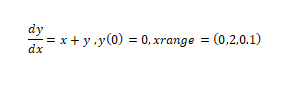```
from scipy.integrate import odeint
import numpy as np
from matplotlib import pyplot as plt
def f(y,x):
return (x +y)
y0 = 0xs = np.arange(0,2,0.1)
ys = odeint(f,y0,xs)
plt.plot(xs,ys,'-')
plt.plot(xs,ys,'ro')
plt.xlabel('x values')
plt.ylabel('y values')
plt.title('Differential equation: dy/dx=x+y')
plt.show()
```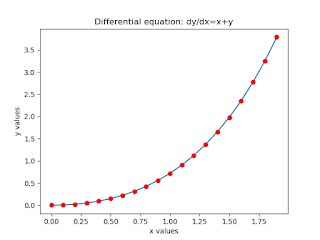```

5. Solving Ordinary differential equation of order first using Eq.from scipy.integrate import odeint
import numpy as np
from matplotlib import pyplot as plt
def f(y,x):
return (3*y-x)
y0 = 0xs = np.arange(0,3,0.1)
ys = odeint(f,y0,xs)
plt.plot(xs,ys,'-')
plt.plot(xs,ys,'ro')
plt.xlabel('x values')
plt.ylabel('y values')
plt.title('Differential equation: dy/dx=(3*y-x)')
plt.show()6. Solving Ordinary differential equation of order first using Eq.from scipy.integrate import odeint
import numpy as np
from matplotlib import pyplot as plt
def f(y,x):
return (x**2)
y0 = 1xs = np.arange(0,5,0.25)
ys = odeint(f,y0,xs)
plt.plot(xs,ys,'-')
plt.plot(xs,ys,'ro')
plt.xlabel('x values')
plt.ylabel('y values')
plt.title('Differential equation: dy/dx=(x**2)')
plt.show()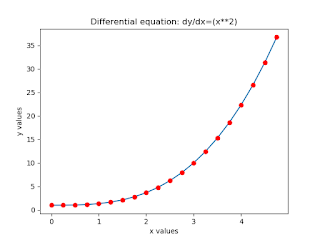```